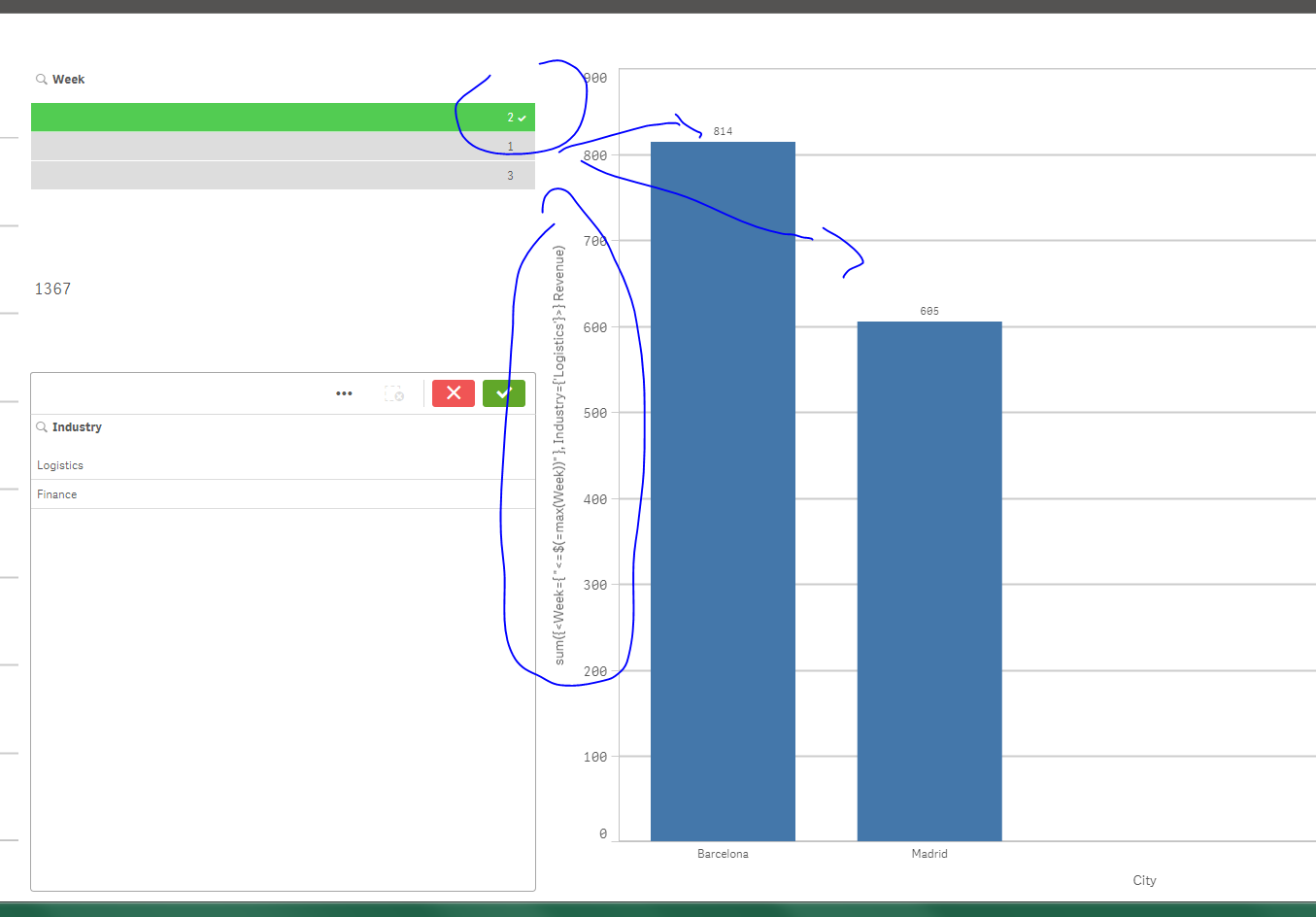# New to Qlik Sense

Discussion board where members can get started with Qlik Sense.

Highlighted
New Contributor III

## Cumulative Sum when a condition is met (Qlik sense)

Dear all,

As may be seen below, we have data of revenue in different cities of Spain and different weeks, coming from different industries. (It's not the real data set, it's just an example)

City            Week        Industry        Revenue

Barcelona  1                Finance        375

Barcelona  1                Logistics      407

Barcelona  2                Finance        375

Barcelona  2                Logistics      407

Barcelona  3                Finance        475

Barcelona  3                Logistics      507

Madrid        1                Finance        280

Madrid        1                Logistics      310

Madrid        2                Finance        290

Madrid        2                Logistics      295

Madrid        3                Finance        290

Madrid        3                Logistics      295

I have a filter with the Week values.  I need to create the following combo chart in qlik sense (all the measures will be displayed as bar charts). As dimension I will have City. One measure should be the cumulative sum (from the first to the maximum week selected on the filter) of revenue in Finance industry and the other measure in Logistics.

On the one hand, I am able to calculate the cumulative sum considering both industries together

(  =Sum({\$<Week={"<=\$(=Max(Week))"}>}[Revenue]}).

On the other hand, I am able to calculate the sum of revenue for each industry

(  =Sum({\$<Industry={"Logistics"}>}[Revenue]}).

I think the solution should be similar to the following code but it does not work:

(  =Sum({\$<Week={"<=\$(=Max(Week))"},Industry={"Logistics"}>}[Revenue]}).

Just as notes:

* Week field is an integer ranging from 1 to 3. It does not come from a date field.

* I cannot use another filter for Industry field because I would like to display the sum of Revenue for each industry separately at any time.

* I have tried to create a calculated field on the Data Manager (Revenue only for Logistics for example) but at the end I'm not obtaining the desired result. In addition, it might not be very efficient with much information. I would like to achieve it only with Set Analysis.

I would really appreciate if anyone could help me solve this issue. Thank you very much in advance.

Best regards,

Gonzalo

Tags (1)
1 Solution

Accepted SolutionsPartner

## Re: Cumulative Sum when a condition is met (Qlik sense)

Try :

sum({<Week={ "<=\$(=max(Week))" }, Industry={'Logistics'}>} Revenue)

I just deleted the last } after Revenue ;

ur expression was like

=Sum({\$<Week={"<=\$(=Max(Week))"},Industry={"Logistics"}>}[Revenue] } )=2 RepliesPartner

## Re: Cumulative Sum when a condition is met (Qlik sense)

Try :

sum({<Week={ "<=\$(=max(Week))" }, Industry={'Logistics'}>} Revenue)

I just deleted the last } after Revenue ;

ur expression was like

=Sum({\$<Week={"<=\$(=Max(Week))"},Industry={"Logistics"}>}[Revenue] } )=New Contributor III

## Re: Cumulative Sum when a condition is met (Qlik sense)

Hi Omar,

I put the extra square bracket only in the community (it was a mistake). Anyway, I started again from scratch following your question and it works.

Thank you very much for your help.

Best regards,

Gonzalo